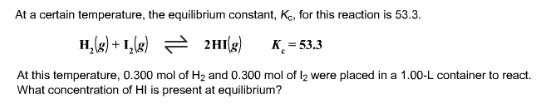# Problem: At a certain temperature, the equilibrium Constant, Ko, for this reaction is 53.3. H2(g) + I2(g) ⇌ 2HI(g) Kc = 53.3 At this temperature, 0.300 mol of H2 and 0.300 mol of I2 were placed in a 1.00-L container to react. What concentration of HI s present at equilibrium?

###### FREE Expert Solution
99% (258 ratings)###### Problem Details

At a certain temperature, the equilibrium Constant, Ko, for this reaction is 53.3. H2(g) + I2(g) ⇌ 2HI(g) Kc = 53.3 At this temperature, 0.300 mol of H2 and 0.300 mol of I2 were placed in a 1.00-L container to react. What concentration of HI s present at equilibrium?